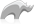##### [ email_compose ]

Method

• Syntax
• Methods
• Traits
``````var(msg) = email_compose(...)
email_immediate(-data=\$msg->data, -from=\$msg->from, -recipients=\$msg->recipients)``````
###### Has methods:
• `email_compose->oncreate(-to = ?, -FROM = ?, -cc = ?, -bcc = ?, -subject = ?, -sender = ?, -replyto = ?, -body = ?, -html = ?, -date = ?, -contentType = ?, -characterset = ?, -transferencoding = ?, -contentdisposition = ?, -headertype = ?, -extramimeheaders = ?, -attachments = ?, -attachment = ?, -htmlimages = ?, -parts = ?)`
• `email_compose->asString()`
• `email_compose->mime_boundary()`
• `email_compose->mime_boundary=(mime_boundary)`
• `email_compose->mime_contenttype()`
• `email_compose->mime_contenttype=(mime_contenttype)`
• `email_compose->mime_hdrs()`
• `email_compose->mime_hdrs=(mime_hdrs)`
• `email_compose->addtextpart(-data = ?, -path = ?)`
• `email_compose->addhtmlpart(-data = ?, -path = ?, -images = ?)`
• `email_compose->addattachment(-data = ?, -name = ?, -path = ?, -type = ?)`
• `email_compose->addpart(-data = ?)`
• `email_compose->data(-prefix::boolean = ?, -force::boolean = ?)`
• `email_compose->recipients()`
• `email_compose->FROM()`
• `email_compose->find(VALUE)`
###### Has traits:
• `any`
• `trait_asString`
• Beginner

### Creating a multi-part message

The code below will create a message with two different text parts and then queue it up to be sent.

Code

``````var(my_msg) = email_compose(-to='to@example.org', -from='from@example.com',
-subject='Re: Subj', -body='Good Deal')
email_queue(-host='smtp.example.com', -data=\$my_msg->data, -from=\$my_msg->from,
-recipients=\$my_msg->recipients)``````Please note that periodically LassoSoft will go through the notes and may incorporate information from them into the documentation. Any submission here gives LassoSoft a non-exclusive license and will be made available in various formats to the Lasso community.